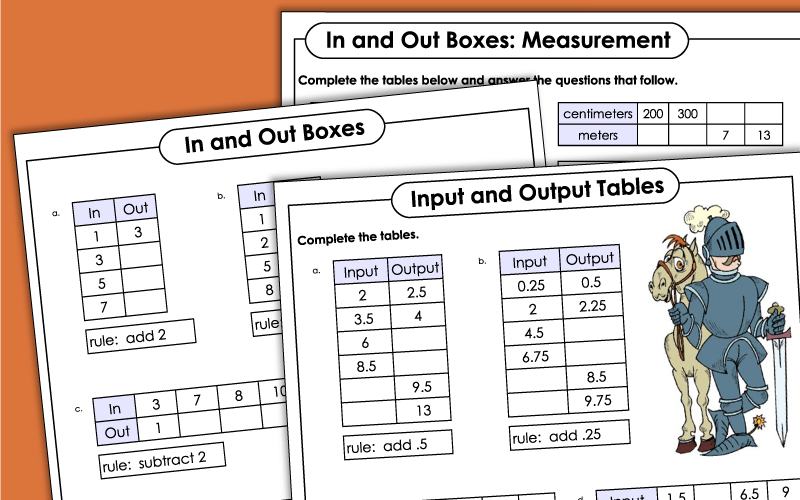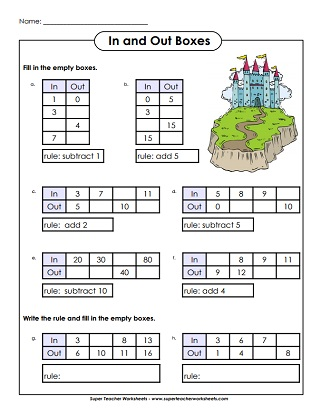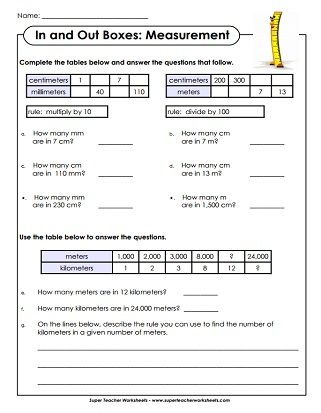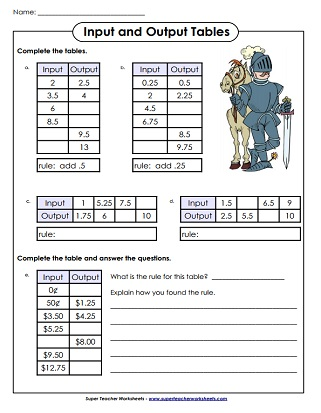# In and Out Boxes

A collection of printable worksheets with addition, subtraction, multiplication, and division input-output tables## Basic

In-out boxes with basic addition and subtraction; Only the out boxes need to be filled in
More in-out boxes with basic addition and subtraction; Students must complete boxes on both sides of the table

## Intermediate

Addition and subtraction in and out boxes; Includes a word problem
Multiplication, division, addition, subtraction in-and-out boxes; Includes a word problem
Two in-out boxes with word problem questions; Addition and subtraction
Input-output boxes for converting linear measurement units; includes word problems; Requires knowledge of multiplication
In-and-out boxes for converting mm, cm, m, and km; includes word problems.

## Also on Super Teacher Worksheets...

Number Pattern Worksheets

Pupils must figure out which numbers come next in each pattern and state the correct rule.

Measurement Worksheets

Practice measuring to the nearest inch, half inch, and quarter inch

## Images of Our WorksheetsMy Account
Site Information